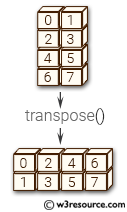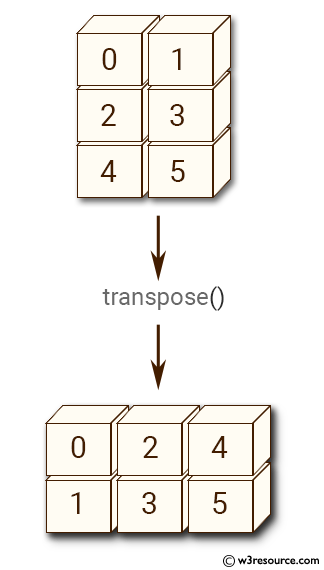# NumPy Array manipulation: transpose() function

## numpy.transpose() function

The transpose() function is used to permute the dimensions of an array.

Syntax:

`numpy.transpose(a, axes=None)`Version: 1.15.0

Parameter:

Name Description Required /
Optional
a Input array. Required
axis By default, reverse the dimensions, otherwise permute the axes according to the values given. Optional

Return value:

[ndarray]: a with its axes permuted. A view is returned whenever possible.

Example-2: numpy.transpose() function

``````>>> import numpy as np
>>> a = np.arange(6).reshape((3,2))
>>> np.transpose(a)
array([[0, 2, 4],
[1, 3, 5]])
``````

Pictorial Presentation:Example-3: numpy.transpose() function

``````>>> import numpy as np
>>> a = np.ones((2,3,4))
>>> np.transpose(a,(1,0,2)).shape
(3, 2, 4)
>>> np.transpose(a,(2,1,0)).shape
(4, 3, 2)
``````

Python - NumPy Code Editor:

Previous: ndarray.T()
Next: Changing number of dimensions atleast_1d()

﻿